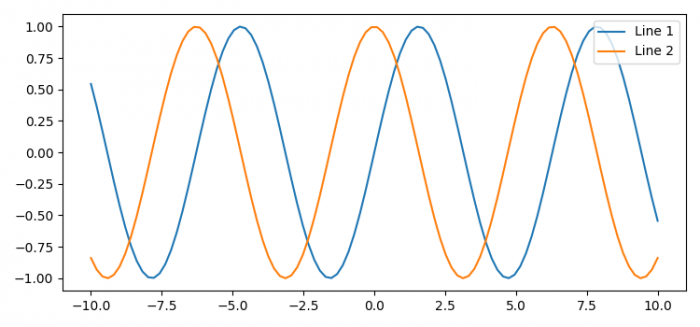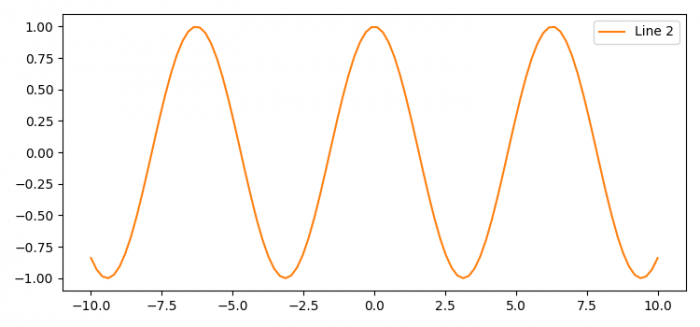# How to hide lines in Matplotlib?

To hide lines in Matplotlib, we can use line.remove() method.

## Steps

• Set the figure size and adjust the padding between and around the subplots.
• Create x, y1 and y2 data points using numpy.
• Make lines, i.e., line1 and line2, using plot() method.
• To hide the lines, use line.remove() method.
• Place a legend on the figure at the upper-right location.
• To display the figure, use show() method.

## Example

import matplotlib.pyplot as plt
import numpy as np

plt.rcParams["figure.figsize"] = [7.50, 3.50]
plt.rcParams["figure.autolayout"] = True

x = np.linspace(-10, 10, 100)
y1 = np.sin(x)
y2 = np.cos(x)

line1, = plt.plot(x, y1, label="Line 1")
line2, = plt.plot(x, y2, label="Line 2")

# line1.remove()

plt.legend(loc="upper right")

plt.show()

## OutputNow, uncomment the line "line1.remove()" and execute the code again. It will produce the following output −